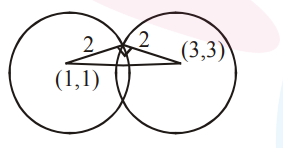# Let C1 and C2 be the centres of the circles`
Question:

let $C_{1}$ and $C_{2}$ be the centres of the circles $x^{2}+y^{2}-2 x-2 y-2=0$ and $x^{2}+y^{2}-6 x-6 y+14=0$ respectively. If $P$ and $Q$ are the points of intersection of these circles, then the area (in sq. units) of the quadrilateral $\mathrm{PC}_{1} \mathrm{QC}_{2}$ is :

1. 8

2. 6

3. 9

4. 4

Correct Option: , 4

Solution:Area $=2 \times \frac{1}{2} \cdot 4=2$